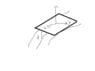# Rotating Coordinates: Solving for x and y

• I
• Nusc
Can you please explain your question some more? How could the answer NOT change if you change the equations and the resulting values?In summary, the conversation discusses the different ways to express a point on a curved surface when rotating a tangent plane. The equations x' = x cos(theta) - y sin(theta) and y' = x sin(theta) + y cos(theta) are used to solve for x and y based on the deviation of the deviation. The question posed is whether the answer would differ if a different point, with equations x' = x cos(theta) + y sin(theta) and y' = - x sin(theta) + y cos(theta), is chosen. The person asking the question does not understand how the answer would not change

#### Nusc

If one rotates a tangent plane on a curved surface, this point can be expressed as follows:

x' = x cos(theta) - y sin(theta)
y' = x sin(theta) + y cos(theta)

One solves for x and y and computes based on the deviation of the deviation.

My question is: would the answer differ if you choose a different point say:

x' = x cos(theta) + y sin(theta)
y' = - x sin(theta) + y cos(theta)

note the negative sign.

Can you please explain your question some more? How could the answer NOT change if you change the equations and the resulting values?

FactChecker said:
Can you please explain your question some more? How could the answer NOT change if you change the equations and the resulting values?
In the attached drawing, I can could approach this new point as

x' = x cos(theta) - y sin(theta)
y' = x sin(theta) + y cos(theta)

or

x' = x cos(theta) + y sin(theta)
y' = - x sin(theta) + y cos(theta)

From what you see in the diagram, how would you justify which coordinates?

#### Attachments

•tangentplane.jpg
9.8 KB · Views: 368
If you cannot, then what should the second pair of coordinates look like visually?

I still don't understand. In the diagram you posted, where are the points (x,y), (x',y'), and the angle theta?